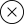# Developmental Math

BNED Courseware for Dev Math provides a bridge between arithmetic and algebra, strengthening arithmetic skills and introducing the student to the fundamental concepts and vocabulary of algebra. The organization of the course makes it easy to adapt to a variety of course syllabi while addressing the needs of students with diverse backgrounds and learning styles. Each topic builds upon previously developed material to demonstrate the cohesiveness and structure of mathematics. Topics include whole numbers, integers, fractions, decimals, percents, real numbers, polynomials, linear equations, using math models, and graphs. Auto-graded activities give students feedback when they are working on their own proving additional data for instructors to hone in on specific areas of weakness for some while helping others with deeper learning opportunities.

Category: .

## Learning Objectives/

#### 1. Whole Numbers

1. 1.1 Identify whole numbers and place values.
2. 1.2 Solve addition problems using whole numbers.
3. 1.3 Solve subtraction problems using whole numbers.
4. 1.4 Solve multiplication problems using whole numbers.
5. 1.5 Solve division problems using whole numbers.

#### 2. The Language of Algebra

1. 2.1 Simplify expressions using order of operations.
2. 2.2 Simplify algebraic expressions.
3. 2.3 Solve equations using addition and subtraction properties of equality.
4. 2.4 Identify multiples and factors of a number.
5. 2.5 Find prime factorization of composite number and Least Common Multiple of two numbers.

#### 3. Integers

1. 3.1 Identify integers on the number line.
2. 3.2 Simplify addition expressions containing integers.
3. 3.3 Simplify subtraction expressions containing integers.
4. 3.4 Simplify multiplication and division expressions containing integers.
5. 3.5 Solve equations using integers.

#### 4. Fractions

1. 4.1 Identify equivalent fractions when given fractions, improper fractions, or mixed numbers.
2. 4.2 Simplify expressions using multiplication and division of fractions.
3. 4.3 Simplify expressions using multiplication and division of mixed numbers and complex fractions.
4. 4.4 Add and subtract fractions with common denominators.
5. 4.5 Add and subtract fractions with different denominators.
6. 4.6 Add and subtract mixed numbers.
7. 4.7 Solve equations containing fractions.

#### 5. Decimals

1. 5.1 Identify decimal place values and corresponding fractional equivalents.
2. 5.2 Perform calculations with decimal numbers.
3. 5.3 Simplify expressions using decimals and fractions.
4. 5.4 Solve equations with decimals.
5. 5.5 Calculate the mean, mode, median of a set of numbers and apply basic definition of probability.
6. 5.6 Solve ratios and rates problems.
7. 5.7 Solve problems with square roots.

#### 6. Percents

1. 6.1 Convert fractions or decimals to percents and percents to fractions or decimals.
2. 6.2 Solve problems using percents.
3. 6.3 Solve sales tax, commission, discount, and mark-up problems involving percents.
4. 6.4 Solve simple interest applications.
5. 6.5 Solve problems using proportions.

#### 7. The Properties of Real Numbers

1. 7.1 Classify rational and irrational numbers.
2. 7.2 Evaluate expressions using the commutative and associative properties.
3. 7.3 Evaluate expressions using the distributive property.
4. 7.4 Evaluate expressions using properties of identities, inverses, and zero.
5. 7.5 Solve problems using systems of measurement.

#### 8. Solving Linear Equations

1. 8.1 Solve equations using the subtraction and addition properties of equality.
2. 8.2 Solve equations using the division and multiplication properties of equality.
3. 8.3 Solve equations with variables and constants on both sides.
4. 8.4 Solve equations with fraction or decimal coefficients.

#### 9. Math Models and Geometry

1. 9.1 Use a problem solving strategy to solve a word or number problem.
2. 9.2 Solve word problems involving coins, tickets, and stamps.
3. 9.3 Solve problems using properties of angles, triangles, and the Pythagorean theorem.
4. 9.4 Solve problems using properties of rectangles, triangles, and trapezoids.
5. 9.5 Solve problems using properties of circles and areas involving irregular shapes.
6. 9.6 Calculate volume and surface area of geometric figures.
7. 9.7 Solve application problems for a specific variable.

#### 10. Polynomials

1. 10.1 Add and subtract monomials and polynomials.
2. 10.2 Simplify expressions using properties of exponents.
3. 10.3 Simplify expressions using multiplication of polynomials.
4. 10.4 Use properties of exponents to divide monomials.
5. 10.5 Solve problems using scientific notation.
6. 10.6 Find greatest common factor to factor polynomials.

#### 11. Graphs

1. 11.1 Use the rectangular coordinate system.
2. 11.2 Graph linear equations by plotting points.
3. 11.3 Use intercepts to graph a line.
4. 11.4 Use slope of a line to solve problems.

There are no reviews yet.

## RELATED COURSES